The simplest representation of a piece of nerve membrane is a simple RC– circuit as shown in Figure 1. The capacitance of a typical membrane, Cm arises due to the fact that there are layers of conductive and nonconductive (lipids) media. The capacitance of a typical patch of membrane isThat is the membrane capacitance is measured in terms of the area of the membrane. The larger the area, the larger the capacitance. Since we will be doing most measurements in microns but most constants are in centimetres, the conversion factor is 104 microns per centimeter. The actual capacitance is then CMA where A is the area of the membrane patch. Thus, a spherical cell which is 20 microns in diameter has a total capacitance of

Figure 1: Passive Membrane Model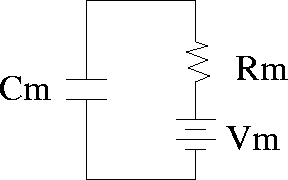The membrane also has an associated resistance. As you might guess, the smaller the patch of membrane, the larger is the resistance. The resistance of a typical patch of membrane, RM is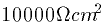so that for our sphere, the actual resistance is Rm=RM/ A or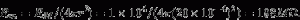There are two main points to emphasize:

(i) Associated with any membrane are certain material constants that are independent of the shape of the membrane,

(ii) the actual electrical properties of a membrane depend on its geometry.

We can now write the equation for a patch of membrane: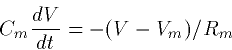(3)

which, if we multiply both sides by Rm obtain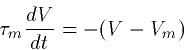(4)

where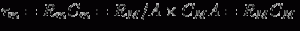is called the membrane time constant. It is independent of geometry. For our present choice of parameters,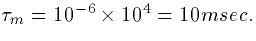The true membrane resistance is difficult to measure since electrodes will puncture the membrane and thus decrease the apparent resistivity. This simple equation is called a one-compartment model for a passive membrane. In general, as we will see, all neural models are made up of pieces just like this and ultimately so are connectionist models that can be derived from these biophysical models. This is the EVE of GENESIS!

The solution to (4) is easy: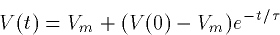where V(0) is the initial voltage.

We could apply a steady current to this membrane as well. Typically, currents are measured in terms of the area of membrane stimulated or current density. The units are typically microamperes per centimeter. This is convenient since one micro-farad times one milli-volt per one milli-second gives one micro-ampere. Positive currents are inward relative to the cell and are called depolarizing while negative currents which are outward relative to the cell are called hyperpolarizing. Suppose we take Vm=-70mV and apply a step of current to our spherical cell. Let’s apply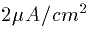. What is the voltage as a function of time? The equation is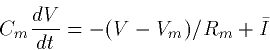(5)

whose solution is again easy to obtain if I is constant:

(6)

Thus the voltage rises or falls to a new value dependent on the current density. The current,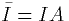where I is the current density. Note that the final value of the potential is dependent on the current density and not the actual geometry of the cell since the final potential is

For our present example, the steady state voltage is

Note the factor of 1000 is necessary to convert to millivolts.

HOMEWORK

1. Calculate Rm and Cm for a cylindrical cell with diameter of 10 microns and length of 50 microns. Ignore the area at the ends of the cylinder.
2. How much current is needed to raise the potential to -55 mV in the spherical cell example above?
3. Suppose that RM is. What is the time constant of the membrane.
4. Suppose that the spherical cell above is initially at rest. Write the equation for the voltage as a function of time if the current is stepped up to 10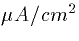for 20 msec and then set to 0 again. (HINT: Use (6) twice, once for the time when the stimulus is on. Then again, using the voltage at the end of the stimulus as initial voltage.) Sketch the voltage response to this current pulse.